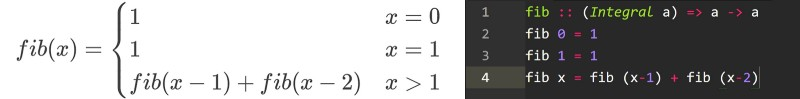by Andrea Zanin

# How a purely functional programming language can change your life.

I believe everyone should learn Haskell, even if you won’t use it in your work. It’s beautiful, and it changes the way you think.

Introductions first: what is Haskell? Haskell is a lazy, purely functional programming language.

What’s that now?

Well, lazy means that Haskell will not execute your commands right away, but will wait until you need the result. At first this may seem strange, but it allows for some pretty nice features — like infinite lists:

``evenNumbers = [0, 2..]``

This snippet will declare an array containing all the even numbers. But as we said, Haskell is lazy so it won’t compute anything until forced to do so.

``take 10 evenNumbers``

The code returns the first 10 elements of evenNumbers, so Haskell will only compute those.

Bonus: as you can see, in Haskell you call a function without parenthesis. You just enter the function’s name followed by the arguments (as in the terminal, if you please).

We also said that Haskell is purely functional. This means that, in general, functions have no side effects. They are black boxes that take input and spit an output without affecting the program in any other way.

Bonus: This makes testing much easier, because you don’t have some mysterious state that is going to break your function. Whatever your function needs is passed as an argument and can be tested.

#### Math, recursion, and Haskell enter a bar

I would also add that Haskell is really like math. I’ll explain myself with an example: the Fibonacci sequence.Fibonacci sequence as defined in math and Haskell. Haskell version is not optimized at all

As you can see, the definitions are very similar. Too similar you may say.

So where are the loops?

You don’t need them! Those four lines are all it takes in Haskell to calculate the Fibonacci sequence. It’s almost trivial. It’s a recursive definition, meaning that the function calls itself. For the sake of comprehension, here is an example of a recursive function:

``factorial :: (Integral a) => a -> afactorial 0 = 1factorial x = x * factorial (x-1)``

Here is what the computer does when calculating the call factorial 5:

``factorial 5 = 5 * factorial 4factorial 4 = 4 * factorial 3factorial 3 = 3 * factorial 2factorial 2 = 2 * factorial 1factorial 1 = 1 * factorial 0factorial 0 = 1``
``factorial 1 = 1 * 1 = 1factorial 2 = 2 * 1 = 2factorial 3 = 3 * 2 = 6factorial 4 = 4 * 6 = 24factorial 5 = 5 * 24 = 120``

You may think that this approach is inefficient, but that’s not true. With some care you can reach C-like speed, sometimes even slightly better (see this stackoverflow thread for more).

#### Wait! Did you say no variables?

Yes, Haskell has no variables — just constants. Well OK, in theory Haskell has variables. But you rarely use them.

How can this be? You cannot code without variables, that’s nuts!

Well, most languages are imperative. This means that most of the code goes towards explaining to the computer how to execute some task. Haskell, on the other hand, is declarative. So most of you code goes into defining the result you want (constants ≈ definitions). Then the compiler will figure out how to do it.

As we already discovered, functions in Haskell are pure. There is no state to modify, and no need for variables. You pass data through various functions and retrieve the final result.

#### Type system (no I’m not going into the static vs dynamic debate)

While learning Haskell’s type system, the first jaw-dropper for me was algebraic data types. At first sight, they’re a bit like enums.

``data Hand = Left | Right``

We just defined a Hand data type that can take the value Left or Right. But let’s see a slightly more complex example:

``data BinTree = Empty          | Leaf Int          | Node BinTree BinTree``

We are defining a binary tree, using a recursive type. Type definitions can be recursive!